Home | | Maths 11th std | Exercise 2.13: Choose the correct or the most suitable answer

# Exercise 2.13: Choose the correct or the most suitable answer

Multiple choice questions with answers / choose the correct answer with answers - Maths Book back 1 mark questions and answers with solution for Exercise Problems - Mathematics : Basic Algebra

CHAPTER: Basic Algebra

Choose the correct or the most suitable answer.

1. If |x + 2| ≤  9, then x belongs to

(1) (-∞,-7)

(2) [-11, 7]

(3) (-∞,-7) [11, ∞)

(4) (-11, 7)

Solution2. Given that x, y and b are real numbers x < y, b > 0, then

(1) xb < yb

(2) xb > yb

(3) xb ≤ yb

(4) x/b ≥ y/b

Solution3. If |x 2| / (x 2) ≥ 0, then x belongs to

(1) [2, ∞)

(2) (2, ∞)

(3) (-∞, 2)

(4) (-2, ∞)

Solution4. The solution of 5x - 1 < 24 and 5x + 1 > -24 is

(1) (4, 5)

(2) (-5,-4)

(3) (-5, 5)

(4) (-5, 4)

Solution5. The solution set of the following inequality |x - 1| ≥ |x 3| is

(1) [0, 2]

(2) [2,∞)

(3) (0, 2)

(4) (-∞, 2)

Solution6. The value of log√2 512 is

(1) 16

(2) 18

(3) 9

(4) 12

Solution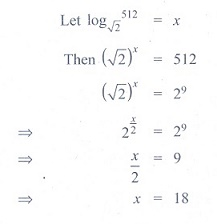7. The value of log31/81 is

(1) -2

(2) -8

(3) -4

(4) -9

Solution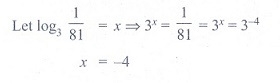8. If log√x 0:25 = 4, then the value of x is

(1) 0:5

(2) 2:5

(3) 1:5

(4) 1:25

Solution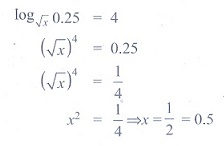9. The value of loga b logb c logc a is

(1) 2

(2) 1

(3) 3

(4) 4

Solution10. If 3 is the logarithm of 343, then the base is

(1) 5

(2) 7

(3) 6

(4) 9

Solution11. Find a so that the sum and product of the roots of the equation 2x2 + (a - 3)x + 3a - 5 = 0 are equal is

(1) 1

(2) 2

(3) 0

(4) 4

Solution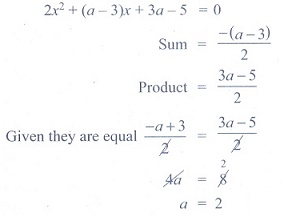12. If a and b are the roots of the equation x2 - kx + 16 = 0 and satisfy a2 + b2 = 32, then the value

of k is

(1) 10

(2) -8

(3) -8, 8

(4) 6

Solution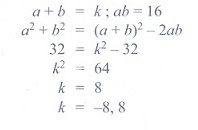13. The number of solutions of x2 + |x 1| = 1 is

(1) 1

(2) 0

(3) 2

(4) 3

Solution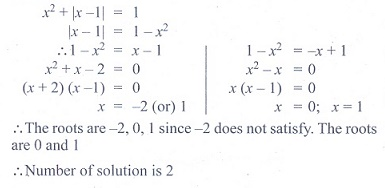14. The equation whose roots are numerically equal but opposite in sign to the roots of 3x2 - 5x - 7 = 0 is

(1) 3x2 - 5x - 7 = 0

(2) 3x2 + 5x - 7 = 0

(3) 3x2 - 5x + 7 = 0

(4) 3x2 + x - 7

Solution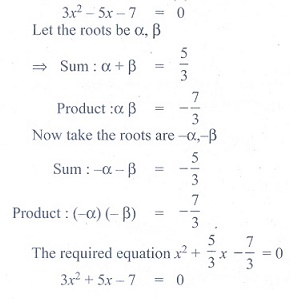15. If 8 and 2 are the roots of x2 +ax+c = 0 and 3, 3 are the roots of x2 +dx+b = 0, then the roots

of the equation x2 + ax + b = 0 are

(1) 1, 2

(2) -1, 1

(3) 9, 1

(4) -1, 2

Solution16. If a and b are the real roots of the equation x2 - kx + c = 0, then the distance between the points (a, 0) and (b, 0) is

(1) √(k2 - 4c)

(2) √(4k2 c)

(3) √(4c - k2)

(4) √(k - 8c)

Solution17. If, then the value of k is

(1) 1

(2) 2

(3) 3

(4) 4

Solution18. If, then the value of A + B is

(1) -1/2

(2) -2/3

(3) 1/2

(4) 2/3

Solution19. The number of roots of (x + 3)4 + (x + 5)4 = 16 is

(1) 4

(2) 2

(3) 3

(4) 0

Solution20. The value of log3 11. log11 13. log13 15.log15 27.log27 81 is

(1) 1

(2) 2

(3) 3

(4) 4

SolutionTags : Basic Algebra | Mathematics , 11th Mathematics : UNIT 2 : Basic Algebra
Study Material, Lecturing Notes, Assignment, Reference, Wiki description explanation, brief detail
11th Mathematics : UNIT 2 : Basic Algebra : Exercise 2.13: Choose the correct or the most suitable answer | Basic Algebra | Mathematics